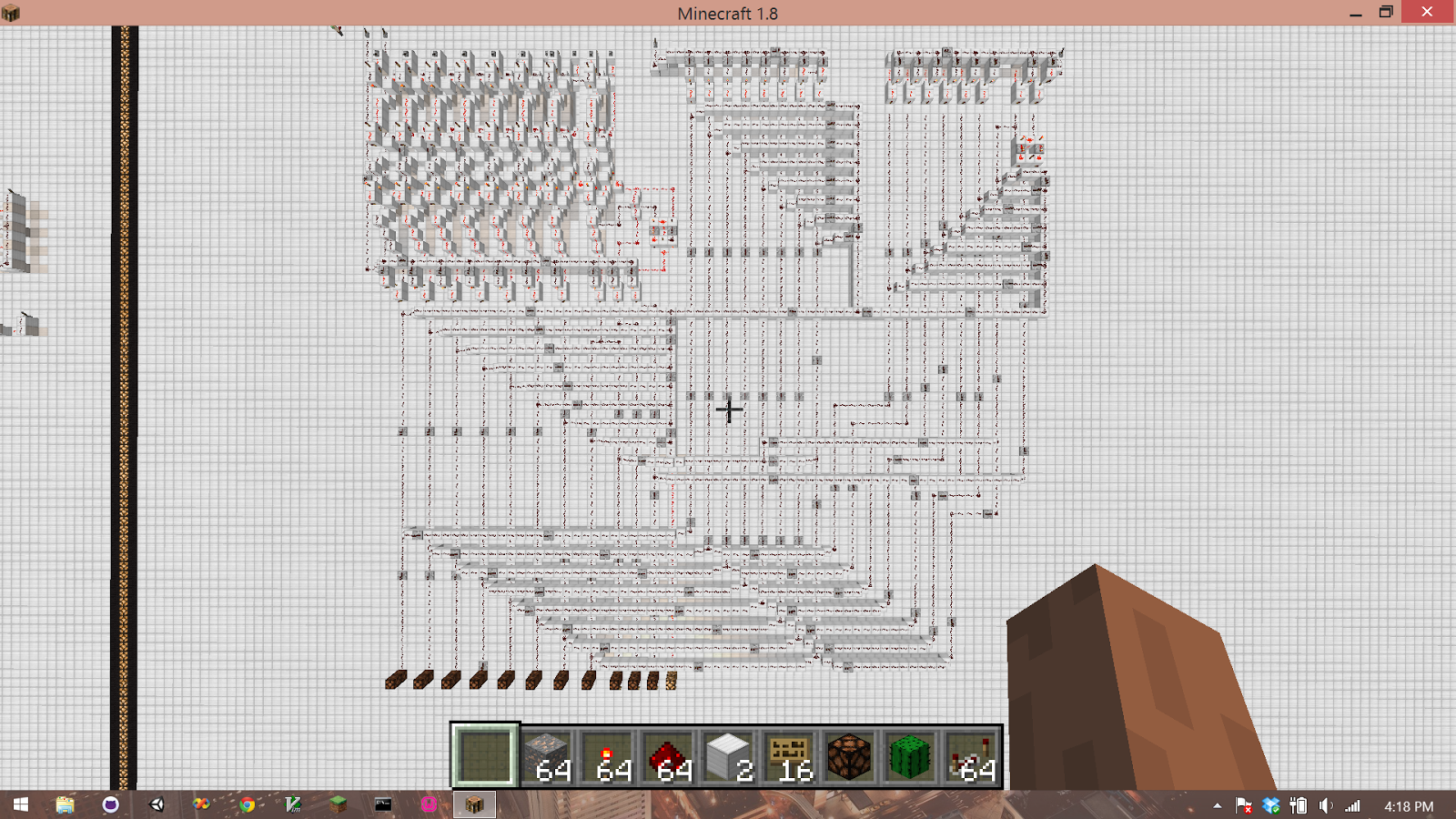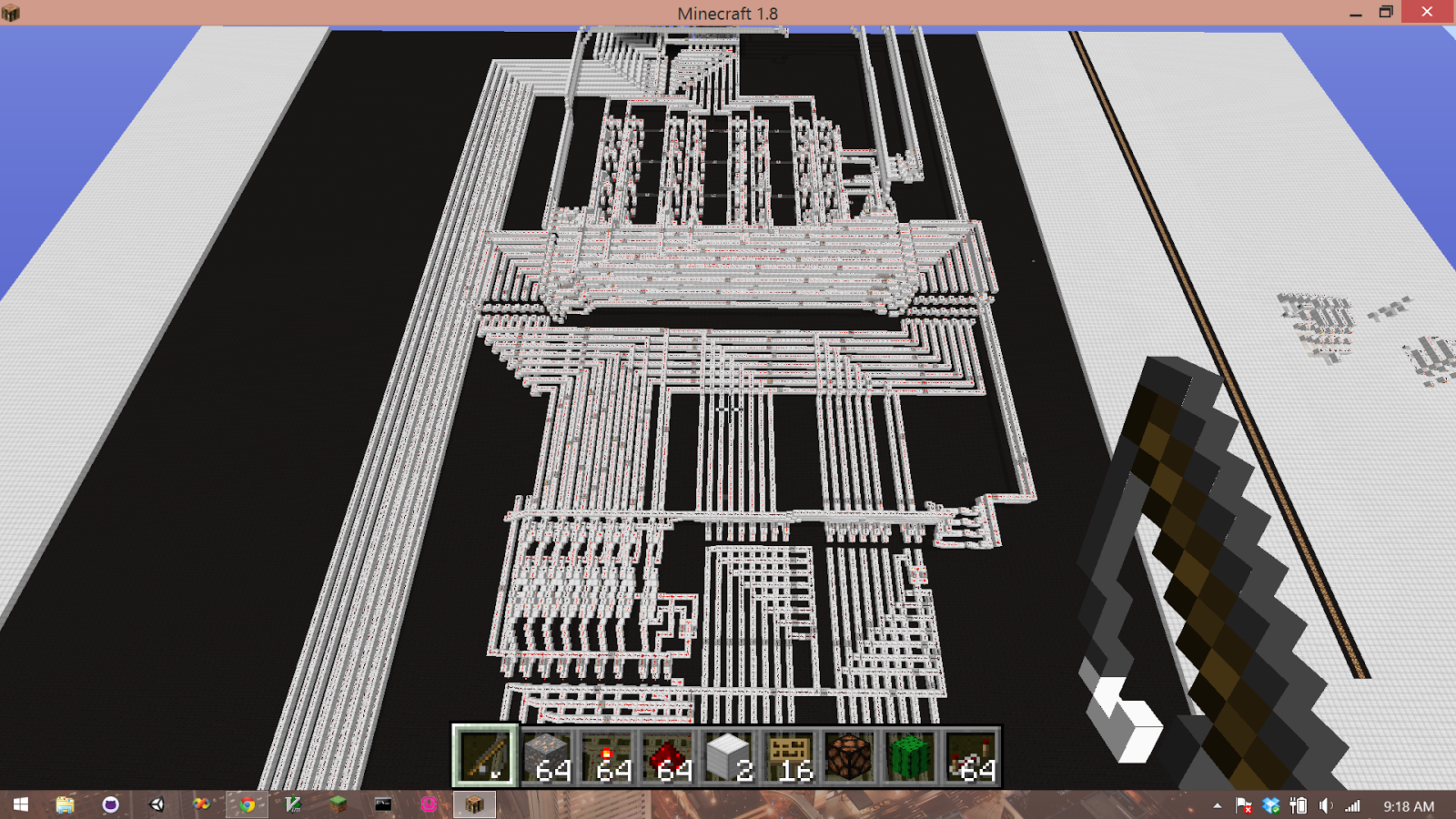Minecraft ALU

CSC312 Fall 2014

Jeffrey Hartman# Overview

The Arithmetic Logic Unit (ALU) can be thought of as the “number crunching” component of your computer and is a key part of the Central Processing Unit.  Even though the ALU in your computer is capable of performing much more complicated operations than the one pictured above, simulations in Minecraft still provide interesting insights into the inner workings of a computer.

The above ALU takes two 8-bit words as input and can perform four different operations: add (A+B), subtract (A-B), arithmetic shift left (2A) or arithmetic shift right (A/2).  Each operation has an enable line that can be selected using a decoder.

# Output

The Redstone Lamps at the bottom of the picture are the output bits of the ALU.  Starting from the left, the first 8 lamps represent the 8-bit result of the operation.  The remaining 4 lamps are the flag bits: negative (N), carry (C), overflow (V) and zero (Z).

# The result word

The result word is a two’s complement binary number.  The range of numbers that can be represented is from -128 (represented by 1000 0000 in 8-bit two’s complement) through 127 (represented by 0111 1111 in 8-bit two’s complement).

# Negative

The negative bit is set if the most significant bit of the result is also set.  This is because the most significant bit of the answer also represents the sign of the number in two’s complement.

# Carry

The carry flag will be set if there is a carry left over from an addition operation.  For example:

1111

1111 0000

+ 0111 0000

0110 0000

In base 10, this example is -16 + 112 = 96. Notice the final 1 that doesn’t fit into the 8-bits.  In this example the carry bit would be set to indicate that we are carrying a 1 into the 9th position.

# Overflow

## In the ALU

Overflow occurs when adding two positive numbers yields a negative result or when adding two negative numbers yields a positive result.  Overflow is detected if the carry in to the sign bit and the carry out of the sign bit are different.  In other words, the carry in to the sign bit and the carry out of the sign bit go through an XOR gate which controls whether or not the overflow bit is set.  Recall that an XOR gate yields true only when the inputs are different.  Consider the following example:

111

0111 0000

+ 0011 0000

1011 0000

In base 10, this example is 112 + 48 = -80.  This is a case where adding two positive numbers yields a negative result.  The answer is not valid and in this case the overflow bit would be set to indicate this condition.

## In shift left

I implemented multiplication by two through arithmetic shift left.  This operation has potential to produce an overflow condition.  Overflow in arithmetic shift left occurs when the two most significant bits are different.  Consider the following example as an illustration:

0101 0000        (80)

<<<<<<<<<

1010 0000        (-96)

Because the two most significant bits are different, the sign of the number has changed and overflow has occurred.

Zero

The zero flag is simply the negation of the result bits.  In other words, the zero flag will be set if and only if all of the output bits are zero.

# Conclusions

The amount of computer hardware simulations possible in Minecraft is astounding.

With some collaboration between Dr. Thorne and other students, we were able to connect the ALU to four 8-bit registers:I suggest building circuits in Minecraft for anyone who wants to learn about computer hardware at the logic gate level.  Using circuits that you’ve built yourself in Minecraft will also create a deeper appreciation for the speed and power of modern computers.

If you’re interested in trying out the circuits for yourself, you can download a copy of the Minecraft world here:

http://gchpcc.georgetowncollege.edu/~dthorne0/20141210_02_flat0000.zip

Instructions for adding a world to your single player mode can be found here: